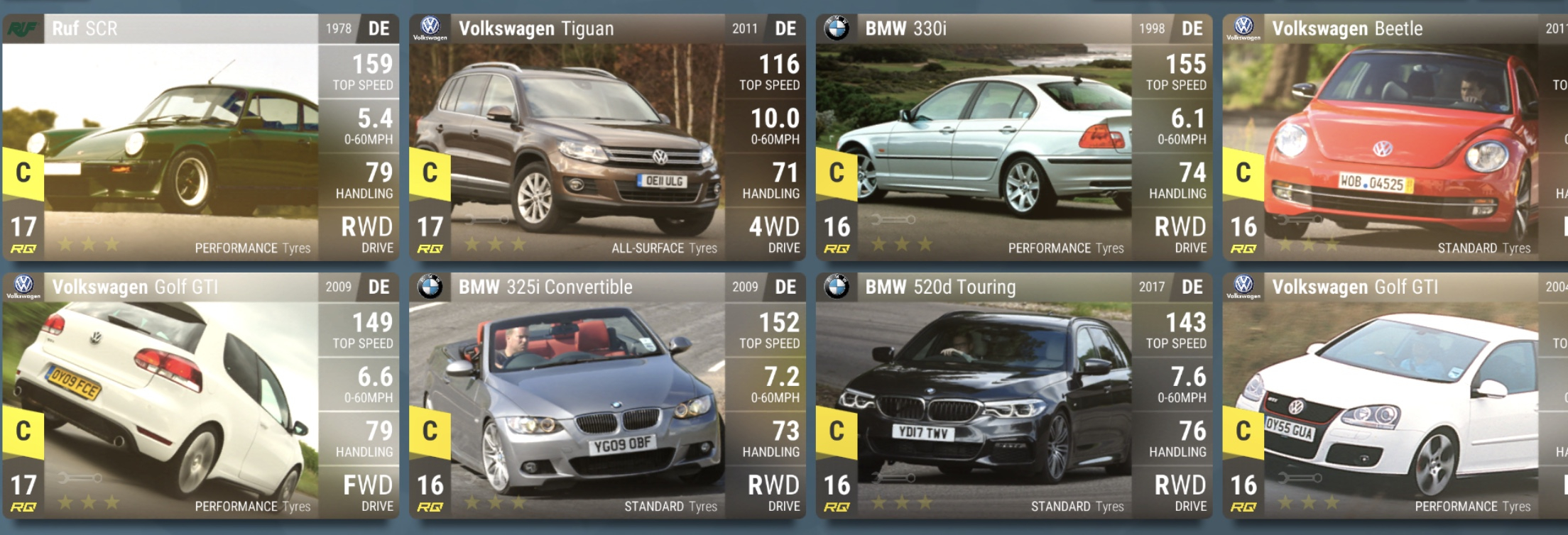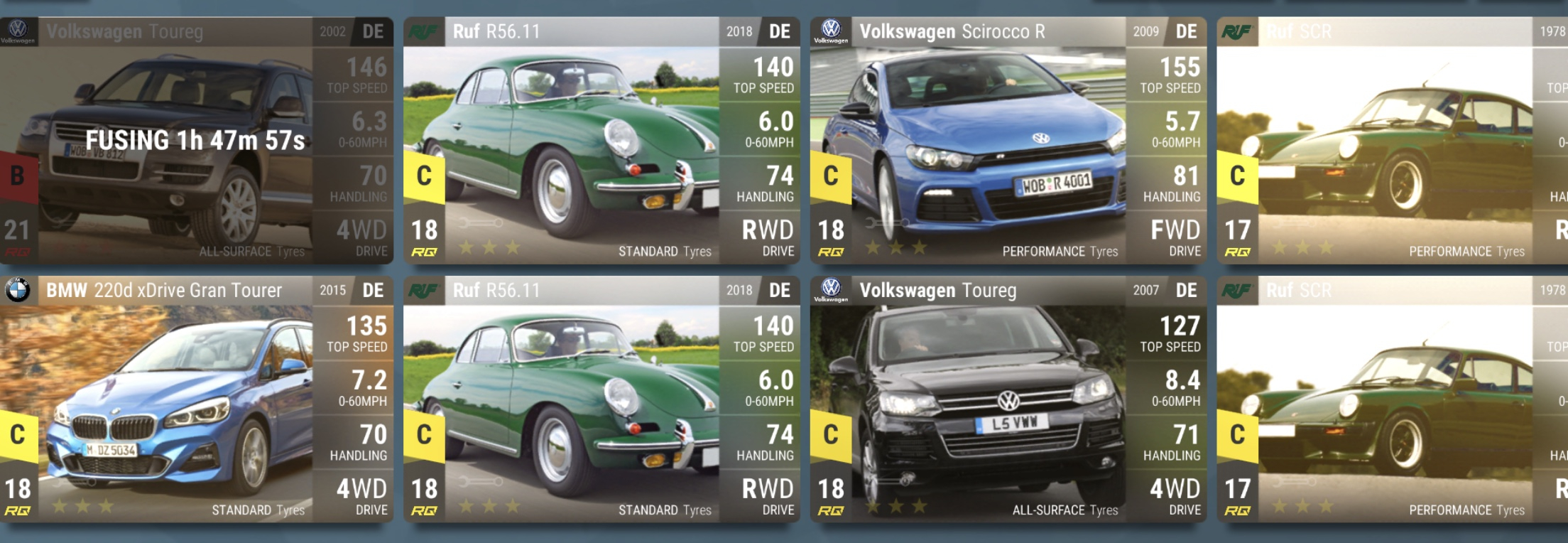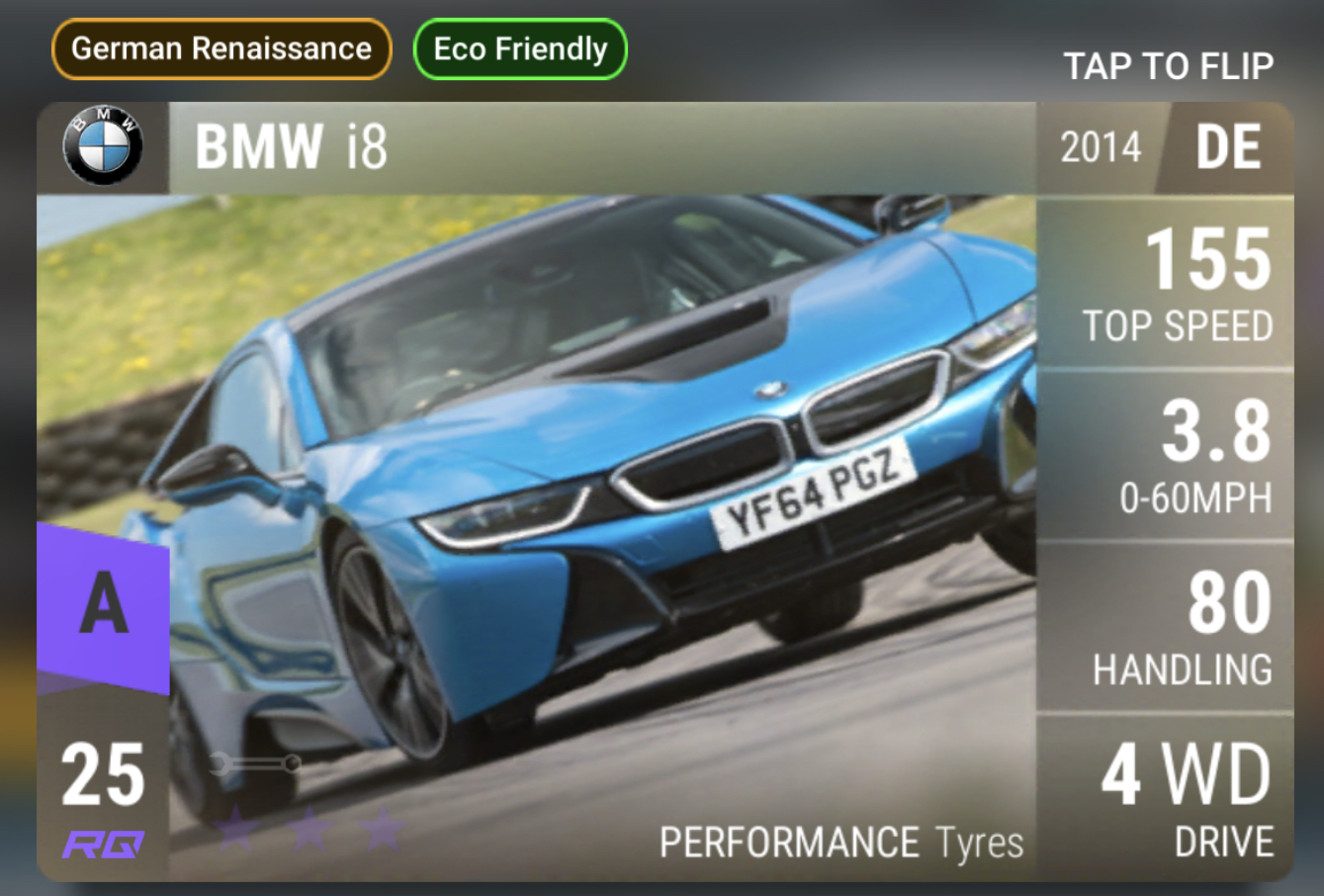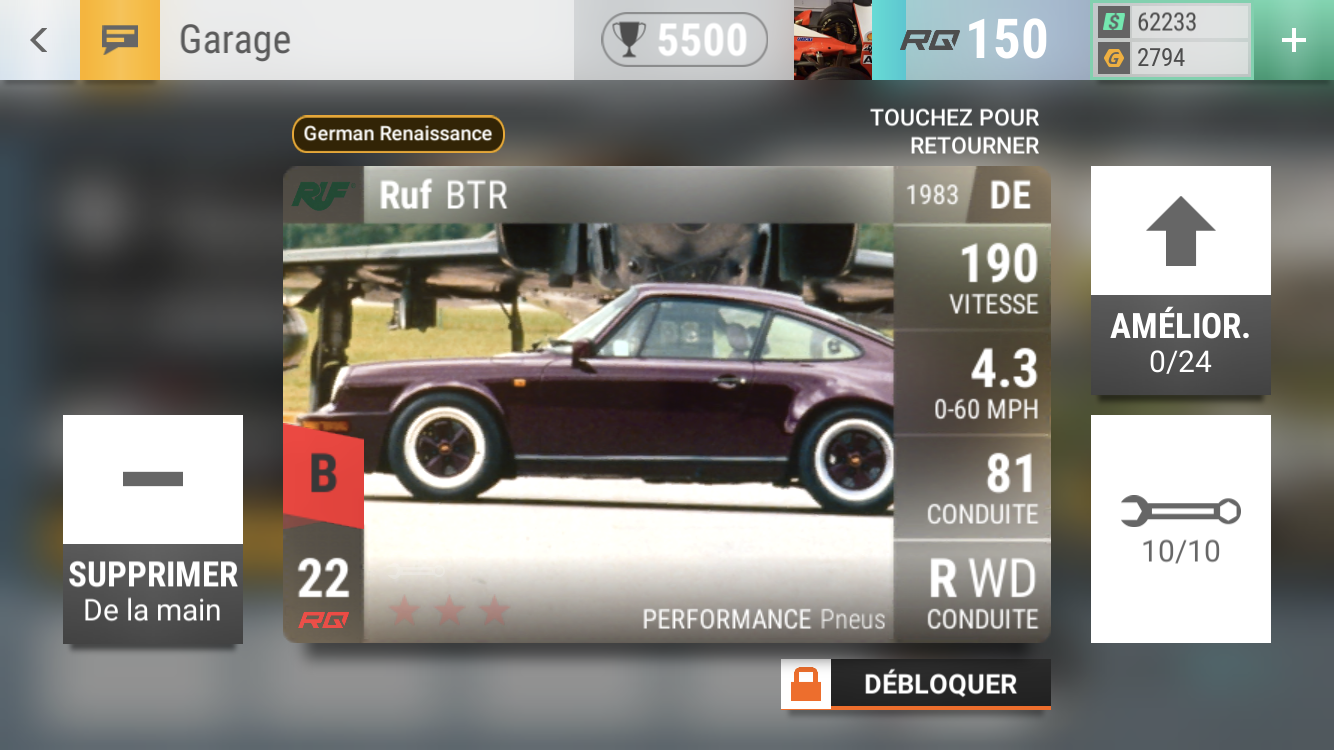# Decent pulls... All my S and A recent pulls

• Lol i just did excactly the same and got this minutes ago. guess we catched the Audi giveaway (randomness?)You probably won't get to use it anyways lol. I have 2 and they collect dust
• edited June 24
This from German premiums made me happy enough (\$1.5)This from a ceramic made me ecstatic!• This from German premiums made me happy enough (\$1.5)This from a ceramic made me ecstatic!Those C class RUFs are going to be fuego
• lg29 said:
This from German premiums made me happy enough (\$1.5)This from a ceramic made me ecstatic!Those C class RUFs are going to be fuego
The RUF is on fire
• I've found this monster. Any idea how to max?• I've found this monster. Any idea how to max?I would go 303, but try to test MRA before finishing it this way.
• This from German premiums made me happy enough (\$1.5)This from a ceramic made me ecstatic!I'm also thinking that the i8 should be on STD tyres as it runs eco-rubber.  I can't find the actual OEM tyres, does anyone know?  It would be a beast in the rain if it was.
• Antoun said:
I've found this monster. Any idea how to max?I would go 303, but try to test MRA before finishing it this way.
Updated 2.3 2.0 2.3 does 0-100 in 7.79s.
•mra 71.4
• S3XY said:mra 71.4
Soooo it's basically a potato
• S3XY said:mra 71.4
Soooo it's basically a potato
Yeah. rq27 might be accurate to describe how bad it is.
The only use of this car I can imagine is to scare opponents away, but it needs to be accompanied by some other Legendaries.
• S3XY said:mra 71.4
I m sorry to ask this maybe a dumb question, but how do you calculate the MRA?
• Anzatoro said:
S3XY said:mra 71.4
I m sorry to ask this maybe a dumb question, but how do you calculate the MRA?
100 * (acc0_60 / (acc0_100 - acc0_60)) so if your car's 0-60 is 3.8 and the 0-100 is 9.2 you can calculate it with this formula 100*(3.8/(9.2-3.8))=70.37 which means your car's MRA is 70.37
• DING097 said:
Anzatoro said:
S3XY said:mra 71.4
I m sorry to ask this maybe a dumb question, but how do you calculate the MRA?
100 * (acc0_60 / (acc0_100 - acc0_60)) so if your car's 0-60 is 3.8 and the 0-100 is 9.2 you can calculate it with this formula 100*(3.8/(9.2-3.8))=70.37 which means your car's MRA is 70.37
If this formula is reliable then the MRA for the RUF BTR should be around 107. Which is insane for a B-Class car with these kind of stats:• edited June 26
lg29 said:
DING097 said:
Anzatoro said:
S3XY said:mra 71.4
I m sorry to ask this maybe a dumb question, but how do you calculate the MRA?
100 * (acc0_60 / (acc0_100 - acc0_60)) so if your car's 0-60 is 3.8 and the 0-100 is 9.2 you can calculate it with this formula 100*(3.8/(9.2-3.8))=70.37 which means your car's MRA is 70.37
If this formula is reliable then the MRA for the RUF BTR should be around 107. Which is insane for a B-Class car with these kind of stats:You might be doing it in kmh? I got to 81.13 doing it with mph
• TD42792 said:
lg29 said:
DING097 said:
Anzatoro said:
S3XY said:mra 71.4
I m sorry to ask this maybe a dumb question, but how do you calculate the MRA?
100 * (acc0_60 / (acc0_100 - acc0_60)) so if your car's 0-60 is 3.8 and the 0-100 is 9.2 you can calculate it with this formula 100*(3.8/(9.2-3.8))=70.37 which means your car's MRA is 70.37
If this formula is reliable then the MRA for the RUF BTR should be around 107. Which is insane for a B-Class car with these kind of stats:You might be doing it in kmh? I got to 81.13 doing it with mph

How can he do it in kmh? The acceleration on the card is in mph and also the track is 0-100mph so he cant get a kmh outcome cause all the variables are in mph.
• edited June 26
Anzatoro said:
TD42792 said:
lg29 said:
DING097 said:
Anzatoro said:
S3XY said:mra 71.4
I m sorry to ask this maybe a dumb question, but how do you calculate the MRA?
100 * (acc0_60 / (acc0_100 - acc0_60)) so if your car's 0-60 is 3.8 and the 0-100 is 9.2 you can calculate it with this formula 100*(3.8/(9.2-3.8))=70.37 which means your car's MRA is 70.37
If this formula is reliable then the MRA for the RUF BTR should be around 107. Which is insane for a B-Class car with these kind of stats:You might be doing it in kmh? I got to 81.13 doing it with mph

How can he do it in kmh? The acceleration on the card is in mph and also the track is 0-100mph so he cant get a kmh outcome cause all the variables are in mph.
I dont know, that’s the only explanation I could think of, maybe he converted it!?
• TD42792 said:
Anzatoro said:
TD42792 said:
lg29 said:
DING097 said:
Anzatoro said:
S3XY said:mra 71.4
I m sorry to ask this maybe a dumb question, but how do you calculate the MRA?
100 * (acc0_60 / (acc0_100 - acc0_60)) so if your car's 0-60 is 3.8 and the 0-100 is 9.2 you can calculate it with this formula 100*(3.8/(9.2-3.8))=70.37 which means your car's MRA is 70.37
If this formula is reliable then the MRA for the RUF BTR should be around 107. Which is insane for a B-Class car with these kind of stats:You might be doing it in kmh? I got to 81.13 doing it with mph

How can he do it in kmh? The acceleration on the card is in mph and also the track is 0-100mph so he cant get a kmh outcome cause all the variables are in mph.
I dont know, that’s the only explanation I could think of, maybe he converted it!?
So if it’s 0-60 is 3.1 and it’s 6.0 to 100 is it's MRA 106.89? Maths was never my strong point.
• LittleEnosBurdette said

So if it’s 0-60 is 3.1 and it’s 6.0 to 100 is it's MRA 106.89? Maths was never my strong point.
Yes
• Got it then. 👍🏻
• What is the Twizy’s MRA? Zero?
Or infinity?
• TD42792 said:
Anzatoro said:
TD42792 said:
lg29 said:
DING097 said:
Anzatoro said:
S3XY said:mra 71.4
I m sorry to ask this maybe a dumb question, but how do you calculate the MRA?
100 * (acc0_60 / (acc0_100 - acc0_60)) so if your car's 0-60 is 3.8 and the 0-100 is 9.2 you can calculate it with this formula 100*(3.8/(9.2-3.8))=70.37 which means your car's MRA is 70.37
If this formula is reliable then the MRA for the RUF BTR should be around 107. Which is insane for a B-Class car with these kind of stats:You might be doing it in kmh? I got to 81.13 doing it with mph

How can he do it in kmh? The acceleration on the card is in mph and also the track is 0-100mph so he cant get a kmh outcome cause all the variables are in mph.
I dont know, that’s the only explanation I could think of, maybe he converted it!?
So if it’s 0-60 is 3.1 and it’s 6.0 to 100 is it's MRA 106.89? Maths was never my strong point.
..you're not talking about the BTR anymore, right? It can't possibly make the 0-100 in 6s, even when maxed, can it?
• TD42792 said:
Anzatoro said:
TD42792 said:
lg29 said:
DING097 said:
Anzatoro said:
S3XY said:mra 71.4
I m sorry to ask this maybe a dumb question, but how do you calculate the MRA?
100 * (acc0_60 / (acc0_100 - acc0_60)) so if your car's 0-60 is 3.8 and the 0-100 is 9.2 you can calculate it with this formula 100*(3.8/(9.2-3.8))=70.37 which means your car's MRA is 70.37
If this formula is reliable then the MRA for the RUF BTR should be around 107. Which is insane for a B-Class car with these kind of stats:You might be doing it in kmh? I got to 81.13 doing it with mph

How can he do it in kmh? The acceleration on the card is in mph and also the track is 0-100mph so he cant get a kmh outcome cause all the variables are in mph.
I dont know, that’s the only explanation I could think of, maybe he converted it!?
So if it’s 0-60 is 3.1 and it’s 6.0 to 100 is it's MRA 106.89? Maths was never my strong point.
..you're not talking about the BTR anymore, right? It can't possibly make the 0-100 in 6s, even when maxed, can it?
Sorry no I wasn’t, was just checking how it was done.

• edited June 27
DING097 said:
Anzatoro said:
S3XY said:mra 71.4
I m sorry to ask this maybe a dumb question, but how do you calculate the MRA?
100 * (acc0_60 / (acc0_100 - acc0_60)) so if your car's 0-60 is 3.8 and the 0-100 is 9.2 you can calculate it with this formula 100*(3.8/(9.2-3.8))=70.37 which means your car's MRA is 70.37

Oh oh i just found maybe a flaw in this formula?

How can you multiply and divide decimal numbers with non-decimal time numbers (seconds in particular)? When you multiply or divide the outcome you get is always decimal.

I really dont get it, so the MRA value is just a vague number that shows the power of the car from 60-100mph? Cause its sure not seconds.

Because when you divide lets say 500 with 6.50seconds the outcome is what? If you do this on a calculator it will take 6.50 as SIX POINT FIVE which is 6 and a half. But this is not the case cause 6.50 is 6 seconds and 50 tenths of second...
• Sorry, I may have misunderstood, but 6.50 seconds is 6 and a half.

5 tenths of a second is .5, which is half, right?
• RWare said:
Sorry, I may have misunderstood, but 6.50 seconds is 6 and a half.

5 tenths of a second is .5, which is half, right?
Ohhh you are absolutely right, it is indeed because its a tenth!
I was totally confused because time is not measured in decimals from minutes and up, but it is measured in decimals on seconds and below.

So you actually can multiply 100*6.3 seconds or divide 500/6.2.
• Anzatoro said:
RWare said:
Sorry, I may have misunderstood, but 6.50 seconds is 6 and a half.

5 tenths of a second is .5, which is half, right?
Ohhh you are absolutely right, it is indeed because its a tenth!
I was totally confused because time is not measured in decimals from minutes and up, but it is measured in decimals on seconds and below.

So you actually can multiply 100*6.3 seconds or divide 500/6.2.
Using this definition mra is a unitless value